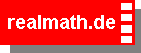Time specification and fractions

You know that there are 60 minutes in one hour.

Now you have to determine the given minutes (min)
as a fraction of one hour (h).

Examples:

 1 h = 1min 60
 1 h = 30min 2
 1 h = 15min 4
 1 h = 12min 5
 3 h = 36min 5
 17 h = 17min 60

Have you figured it out? Try yourself now.### Topic: Fractions

#### Fractions and time

Complete the missing numerator of the fraction!

h =

(c) Andreas Meier, Sophie-Scholl-Realschule Weiden i.d.OPf